# {*no-status title-slide logos} // comment - - ## $whoami {image-full bottom-left darkened /black-bg /no-status} - prob multiple slides {notes} - thesis in software architecture (ambient intelligence) - reorient: postdoc on using probabilistic models for pattern mining: created temporal topic models to unmix activities - reorient: lab H Curien, Saint Étienne, core Machine Learning group (cite names and topics too) - not an expert on most of these stuff ## Overview {#plan .plan image-fit darkened top-right /no-status} - Introduction to Domain Adaptation {da} - Domain Adaptation by Subspace Alignment {dasa} - Landmarks-based Kernelized Subspace Alignment {landmarks} - More? {more} - Contextually Constrained Deep Networks for Scene Labeling - Semantic Scene Parsing Using Inconsistent Labelings # @copy:#plan: %+class:highlight: .da ## Domain Adaptation: What and Why? {libyli} - When do we need Domain Adaptation (DA)? {card} - The training distribution is different from the testing distribution - Example Domain Adaptation task? {card} - Given: labeled images (e.g., from a Web image corpus) - Task: is there a Person in unlabeled images (e.g. from a Video corpus) - {no} - {inlineblock center no custom1} -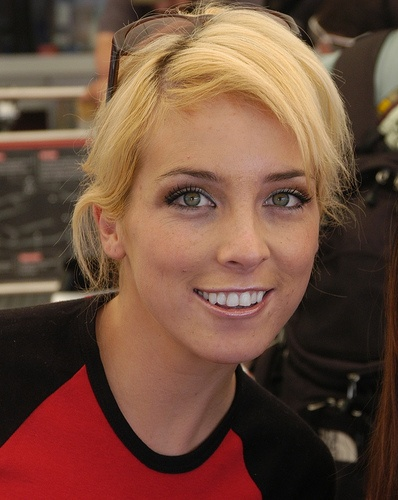Person -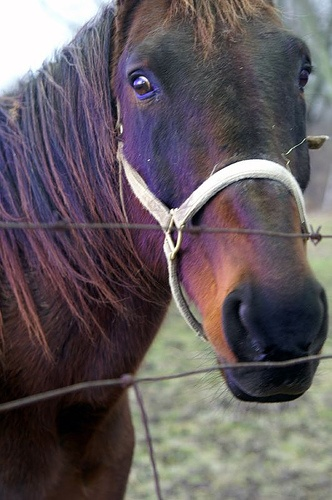not-Person - -Person? -Person? - How can we learn, from one distribution, a low-error classifier on another distribution? ## Domain Adaptation: task and notations {libyli} - Typical binary classification task -$X$: input space,$Y = \\{-1,+1\\} {}$: output space - Typical supervised classification {card} -$\green{P_S} {}$source domain: distribution over$X \times Y$// -$\green{D_S}{}$: marginal distribution over$X$-$\green{S}{} = \\{(x^s_i,y^s_i)\\}_{i=1}^{m_s} \sim (\green{P_S})^{m_s} {}$: a sample of labeled points - Goal: Find a classifier$h \in \mathcal{H}{}$with a low source error$R\_{\green{P\_S}}(h) = \mathbf{E}\_{(x^s,y^s)\sim \green{P_S}}\;\; \mathbf{I}\big[h(x^s)\ne y^s\big] {}$- Domain Adaptation {card} -$\orange{P_T} {}$target domain: distribution over$X \times Y$, ($\orange{D_T}{}$: marginal over$X$) -$\orange{T}{} = \\{(x^t_i)\\}_{j=1}^{m_t} \sim (\orange{D_T})^{m_t} {}$: a sample of unlabeled target points - Goal: Find a classifier$h \in \mathcal{H}{}$with a low target error$R\_{\orange{P\_T}}(h) = \mathbf{E}\_{(x^t,y^t)\sim \orange{P_T}}\;\; \mathbf{I}\big[h(x^t)\ne y^t\big] {}$@svg:images-da/normal-vs-da.svg 375px 280px {normalvsda slide} ## Link the Target Risk to the Source? \begin{matrix} \\\\ R\_{\orange{P\_T}}(h)&=& \mathbf{E}\_{(x^t,y^t)\sim \orange{P\_T}}\mathbf{I}\big[h(x^t)\ne y^t\big]\\\\ \\\\ &=&\mathbf{E}\_{(x^t,y^t)\sim \orange{P\_T}}\frac{\green{P\_S}(x^t,y^t)}{\green{P\_S}(x^t,y^t)}\mathbf{I}\big[h(x^t)\ne y^t\big]\\\\ \\\\ &=&\sum\_{(x^t,y^t)} \orange{P\_T}(x^t,y^t)\frac{\green{P\_S}(x^t,y^t)}{\green{P\_S}(x^t,y^t)}\mathbf{I}\big[h(x^t)\ne y^t\big]\\\\ \\\\ &=&\mathbf{E}\_{(x^t,y^t)\sim \green{P\_S}}\frac{\orange{P\_T}(x^t,y^t)}{\green{P\_S}(x^t,y^t)}\mathbf{I}\big[h(x^t)\ne y^t\big]\\\\ \end{matrix} {latex slide} ## Domain Adaptation − Covariate Shift? {libyli} // This difference between the two domains is called covariate shift (Shimodaira, 2000). - {card dense} - R\_{\orange{P\_T}}(h)\; =\;\; \mathbf{E}\_{(x^t,y^t)\sim \green{P\_S}}\frac{\orange{P\_T}(x^t,y^t)}{\green{P\_S}(x^t,y^t)}\mathbf{I}\big[h(x^t)\ne y^t\big] {latex} - The target risk can be rewritten as an expectation on the source - Covariate Shift {card} - When$\green{P\_S}(y^t|x^t)=\orange{P\_T}(y^t|x^t)$(covariate shift assumption) - Very strong assumption - We can estimate a ratio between unlabeled data - {no} - \begin{matrix} {R\_{\orange{P\_T}}(h)}&=&\mathbf{E}\_{(x^t,y^t)\sim \green{P\_S}}\frac{\orange{D\_T}(x^t)\orange{P\_T}(y^t|x^t)}{\green{D\_S}(x^t)\green{P\_S}(y^t|x^t)}\mathbf{I}\big[h(x^t)\ne y^t\big]\\\\ \\\\ &=&\mathbf{E}\_{(x^t,y^t)\sim \green{P\_S}}\frac{\orange{D\_T}(x^t)}{\green{D\_S}(x^t)}\mathbf{I}\big[h(x^t)\ne y^t\big]\\\\ \end{matrix} {latex no slide} - **⇒ Approach**: density estimation and instance re-weighting {no slide} // actually, it is simpler to estimate the density of the ratio - {notes notslide} - nice pres http://www.slideserve.com/Anita/sample-selection-bias ## Domain Adaptation − Domain Divergence {libyli} - {card dense libyli} - {inlineblock no} - Labeled source samples$S$drawn *i.i.d.* from$\green{P_S}{}${c5} - {c1} - Unlabeled target samples$T$drawn *i.i.d.* from$\orange{P_T}{}${c5} -$h$is learned on the source, how does it perform on the target? - ⇒ it depends on the closeness of the domains{no} - {inlineblock no} --- Adaptation Bound [Ben-David et al., MLJ’10, NIPS’06] {card dense libyli} -$\forall h\in\mathcal{H},\quad R\_{\orange{P\_T}}(h)\ \leq\ \; R\_{\green{P\_S}}(h)\ +\ \frac{1}{2} d\_{\mathcal{H}\;\Delta\;\mathcal{H}}(\green{D\_S},\orange{D\_T})\ +\ \nu $- Domain divergence:$d\_{\mathcal{H}\;\Delta\;\mathcal{H}}(\green{D\_S},\orange{D\_T}) \;=\; 2 \sup\_{(h,h')\in\mathcal{H}^2} \Big| R\_{\orange{D\_T}}(h,h') - R\_{\green{D\_S}}(h,h')\Big| $- Error of the joint optimal classifier:$\nu = \inf\_{h'\in\mathcal{H}}\big(R\_{\green{P\_S}}(h')+R\_{\orange{P\_T}}(h')\big)- {notes notslide} - with some probability (1 - delta) - H a symmetric hypothesis space - More (by M. Sebban), epat slides - about what is d_HH nips 07 # @copy:#plan: %+class:highlight: .dasa ## Unsupervised Visual Domain Adaptation Using Subspace Alignment − ICCV 2013 *Basura Fernando, Amaury Habrard, Marc Sebban, Tinne Tuytelaars (K.U. Leuven)* {paper libyli} - Intuition for unsupervised domain adaptation - principal components of the domains may be shared - principal components should be re-aligned - Principle - extract a source subspace (d$largest eigen vectors) - extract a target subspace ($dlargest eigen vectors) - learn a linear mapping function that aligns the source subspace with the target one {slide anim-continue} -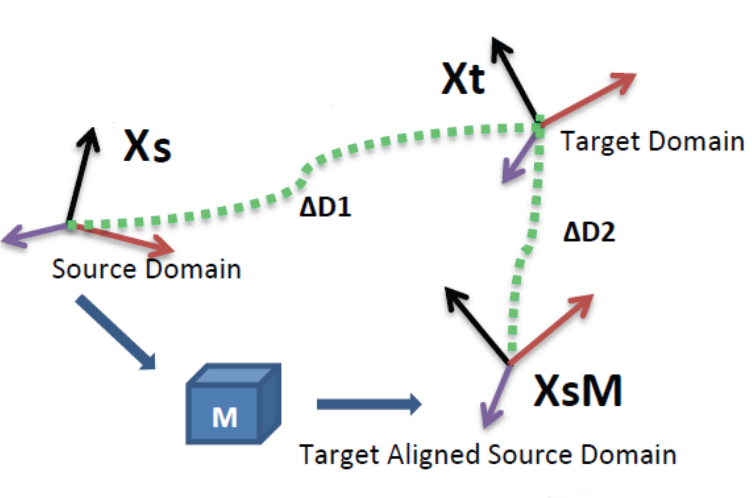{no} - {notes notslide} - KULeuven - More (by M. Sebban) ## Subspace Alignment − Algorithm - Algorithm {card custom2 libyli} - **Input:** Source data\green{S}{}$, Target data$\orange{T}{}$, Source labels$\green{L\_S}{}$- **Input:** Subspace dimension$d${no} - **Output:** Predicted target labels$\orange{L\_T} {}${no} -$\green{X\_S} \leftarrow PCA(\green{S},d)$*(source subspace defined by the first d eigenvectors)* -$\orange{X\_T} \leftarrow PCA(\orange{T},d)$*(target subspace defined by the first d eigenvectors)* -$M \leftarrow \green{X\_S}' \orange{X\_T}{}*(closed form alignment)* -X\_a \leftarrow \green{X\_S} M*(operator for aligning the source subspace to the target one)* -\gray{S\_a} = \green{S} X\_a*(new source data in the aligned space)* -\gray{T\_T} = \orange{T} \orange{X\_T}{}*(new target data in the aligned space)* -\orange{L\_T} \leftarrow Classifier(\gray{S\_a},\green{L\_S}, \gray{T\_T})$- A natural similarity:$Sim(\mathbf{x}\_s,\mathbf{x}\_t)=\mathbf{x}\_sX\_SMX\_T' \mathbf{x}\_t'=\mathbf{x}\_sA \mathbf{x}\_t'{slide} ## Subspace Alignment − Experiments {libyli} - *@svg:images-da-align/img-iccv13.svg 800px 200px* {no} - Comparison on visual domain adaptation tasks // SURF, 800 words? - adaptation from Office/Caltech-10 datasets (four domains to adapt) - adaptation on ImageNet, LabelMe and Caltech-256 datasets: one is used as source and one as target - Other methods - Baseline 1: projection on the source subspace - Baseline 2: projection on the target subspace - 2 related methods: - GFS [Gopalan et al.,ICCV'11] // Geodesic flow subspaces - GFK [Gong et al., CVPR'12] // Geodesic flow kernel ## Subspace Alignment − Results {dense libyli} - Office/Caltech-10 datasets {inlineblock} - @svg:images-da-align/result1-NN-iccv13.svg 330px 250px - @svg:images-da-align/result1-SVM-iccv13.svg 330px 250px - ImageNet (I), LabelMe (L) and Caltech-256 (C) datasets {inlineblock} - @svg:images-da-align/result2-NN-iccv13.svg 330px 130px - @svg:images-da-align/result2-SVM-iccv13.svg 330px 130px ## Subspace Alignment − Recap. {#sarecap} - Good - Very simple and intuitive method - Totally unsupervised - Theoretical results for dimensionality detection - Good results on computer vision datasets - Can be combined with supervised information (future work) - Bad {limitations} - Cannot be directly kernelized to deal with non linearity - Actually assumes that spaces are relatively close - Ugly {limitations} - Assumes that all the source and target examples are relevant - **Idea:** *Select landmarks from both source and target domains to project the data in a common space using a kernel w.r.t those chosen landmarks. Then the subspace alignment is performed. {dense}* {hidden} # @copy:#plan: %+class:highlight: .landmarks # @copy:#sarecap: %+class:highlight: .limitations + %-class:hidden: .hidden ## Principle of Landmarks {libyli} - JMLR 2013 − *Connecting the Dots with Landmarks: Discriminatively Learning Domain-Invariant Features for Unsupervised Domain Adaptation{denser}* {no} - Boqing Gong, Kristen Grauman, Fei Sha - Principle: find source points (the landmarks) such that the domains are similarly distributed “around” {inlineblock} - @svg:images-da-landmarks/landmarks1.svg 280px 100px - @svg:images-da-landmarks/landmarks2.svg 280px 100px - Optimization problem:\min\_\alpha \left\\| \frac{1}{\sum\_m \alpha\_m } \sum\_m \alpha\_m \phi (x\_m) - \frac{1}{N} \sum\_n \phi(x\_n) \right\\|^2${dense} - {no} -$\alpha$: binary landmark indicator variables -$\phi(.)$: nonlinear mapping, maps every$xto a RKHS - minimize the difference in sample-means - \+ a constraint: *labels should be balanced among the landmarks* ## Landmarks-based Kernelized Subspace Alignment for Unsupervised DA − CVPR 2015 *Rahaf Aljundi, Rémi Emonet, Marc Sebban* {paper libyli} - Intuition for landmarks-based alignment - subspace alignment does not handle non-linearity - subspace alignment cannot “ignore” points - landmarks can be a useful to handle locality and non-linearity - Challenges - selecting landmarks in a unsupervised way - choosing the proper Gaussian-kernel scale @svg:images-da-landmarks/landmarks2.svg 700px 200px ## Proposed Approach − Workflow @svg:images-da-landmarks/workflow.svg 700px 400px - @anim: %viewbox:#zlandpro | %viewbox:#zpca | %viewbox:#zalign | %viewbox:#zclassify | %viewbox:#zall - Overall approach - 2 new steps: *landmark selection*, *projection* on landmarks - subspace alignment ## Multiscale Landmark Selection {libyli} - Select landmarks among all points,\green{S} \cup \orange{T} {}$- Greedy selection - consider each candidate point$c$and a set of possible scales$s$- criteria to promote the candidate - after projection on the candidate - the overlap between source and target distributions is above a threshold - Projection: a point is projected with$K(c, p)= \exp \left( \frac{-\left\|c - p\right\|^2}{2 s^2} \right)${dense} - Overlap {libyli} - project source and target points - fit two Gaussians (one for each) -$ overlap(\green{\mu\_S, \sigma\_S} ; \orange{\mu\_T, \sigma\_T}) = \frac{\mathcal{N}(\green{\mu\_S} - \orange{\mu\_T} \mid 0, \sigma\_{sum}^2)}{\mathcal{N}(0 \mid 0, \sigma\_{sum}^2)} $- normalized integral of product - with$\sigma\_{sum}^2 = \green{\sigma\_S}^2 + \orange{\sigma\_T}^2$, and$\mathcal{N}(. \mid 0, \sigma\_{sum}^2)$centered 1d-Gaussian ## Landmark-Based Alignment − Overall {#sadarecap libyli} - Select landmarks among all points,$\green{S} \cup \orange{T} {}$- greedy selection - multi-scale selection - maximize domain overlap - Project all points on the landmarks - use a Gaussian kernel -$\sigma \gets median\\_distance(S \cup T) - Subspace-align the projected points - PCA on source domain - PCA on target domain - compute the alignmentM$## Landmark-Based Alignment − Results {libyli} - Is landmark-based kernelization useful? - *@svg:images-da-landmarks/results.svg 730px 200px* {no} - Is our landmark-selection any good? - *@svg:images-da-landmarks/results-sel-landmarks.svg 730px 200px* {no} # @copy:#plan: %+class:highlight: .more ## Task: Semantic Scene Labeling - For each pixel in an image (or video), predict its class - e.g., building, road, car, pedestrian, sign, ...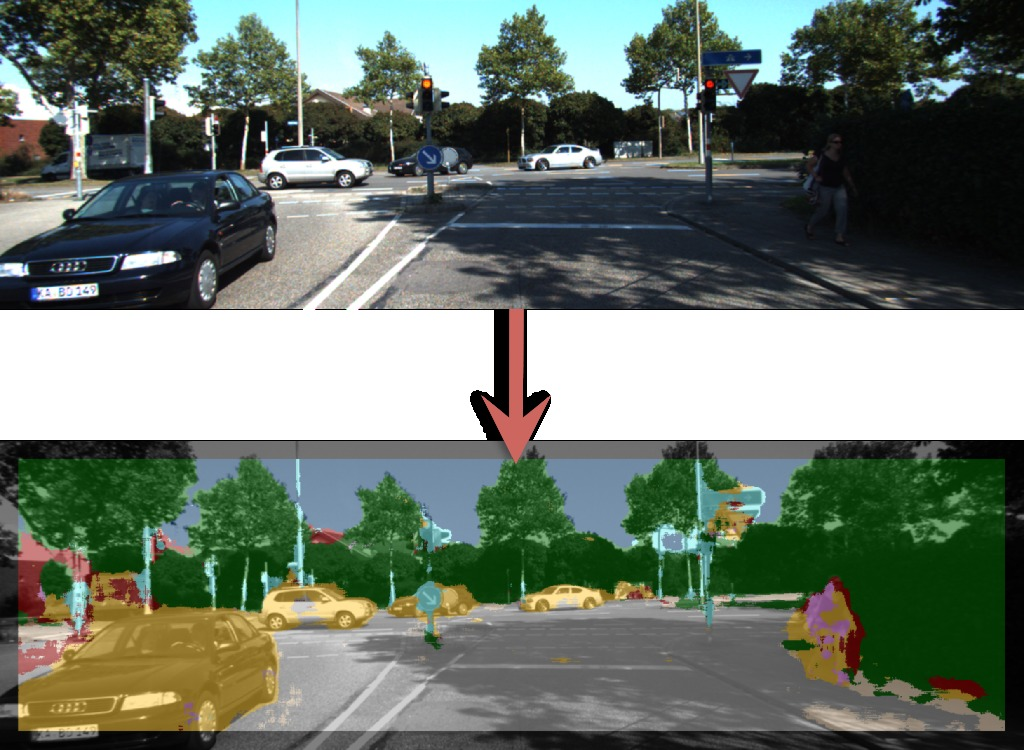## Contextually Constrained Deep Networks for Scene Labeling − BMVC 2015 *Taygun Kekec, Rémi Emonet, Elisa Fromont, Alain Trémeau, Christian Wolf* {paper libyli} - Observation - state of the art uses deep CNN (conv. net.) - learning is patch-based, using the center label - training images are densely labeled - Idea - use labels in the patch to guide the network - force a part of the network to use the context (like an MRF) - @anim: .hasSVG @svg:cnn-context/cnn-app1.svg 800px 200px ## The Network // inspired by Farabet's paper @svg:cnn-context/cnns.svg 800px 400px ## Multi-Step Learning {libyli} @svg:cnn-context/cnn-app1.svg 800px 200px - Learn the context net (yellow) - Learn the dependent net (blue) - freeze the context net - use prediction, mixed with some ground truth (probability$\tau\$) // small - Fine tuning - unfreeze the context net - no intermediate supervision - allow for co-adaptation ## Contextually Constrained − Results @svg:cnn-context/bmvc2014-results.svg 800px 400px ## Semantic Scene Parsing Using Inconsistent Labelings − CVPR 2016?
*Damien Fourure, et al.* {paper libyli} - Context: KITTI dataset - urban scenes recorded from a car - many sensors (RGB, stereo, laser, ...), different tasks - Observation (scene labeling on KITTI) {obs} - different groups labeled frames - they used different frames (mostly) and different labels - the quality/precision of annotations varies - @anim: .obs, .hasSVG - Goal - leverage all these annotations - improve segmentation on individual labelsets/datasets @svg:cnn-context/kitti-amount-labeled.svg 800px 200px ## Labels - 7 different label sets @svg:cnn-context/kitti-labels.svg 800px 200px @svg:cnn-context/kitti-amount-labeled.svg 800px 200px ## First Approach - a) Baseline: separate training - b) Joint Training - with datasetwise soft-max - with selective loss function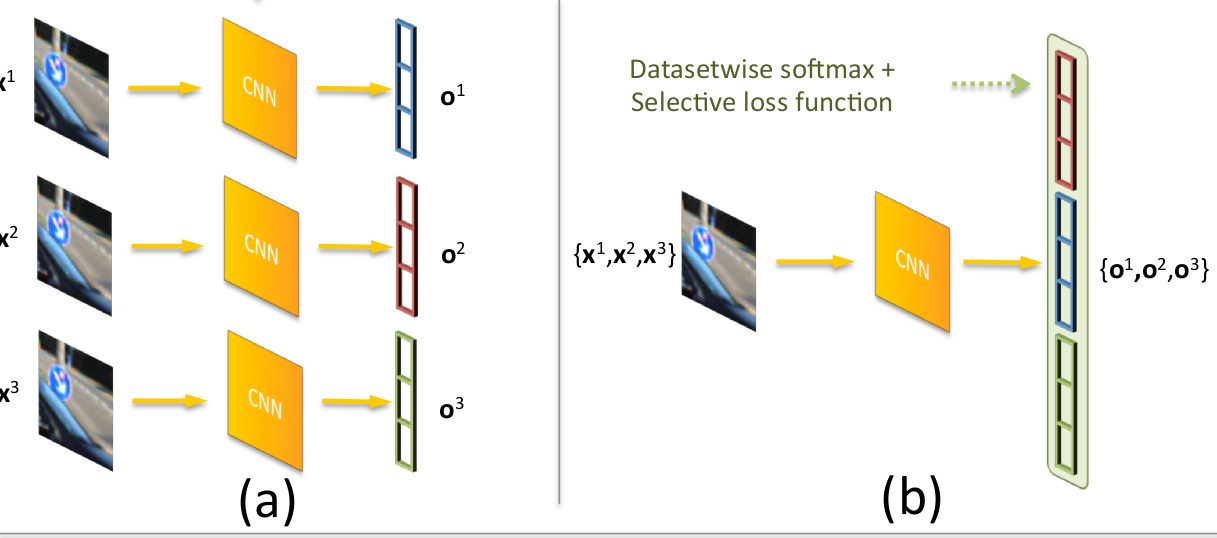## Labels Correlation After Joint Training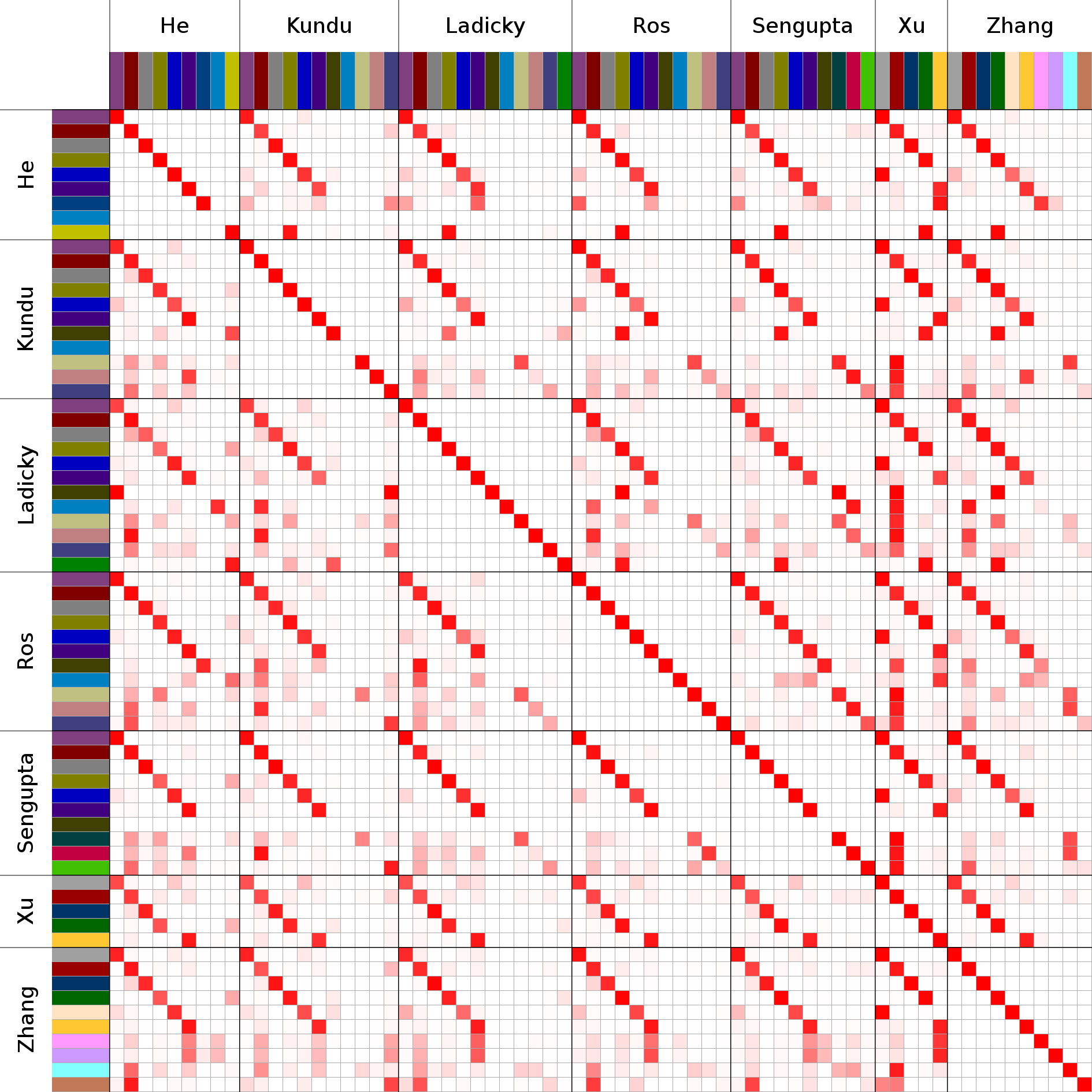- Observing outputs
after joint training {c5 libyli} - correlation across datasets - @anim: img - clear correspondence for some labels - one-to-many correspondences ## Exploiting Correlations after Joint Training - c) Joint Training with shared context {appc} - a single network to learn all correlations - d) Joint Training with individual context {appd} - a specialized network per labeling - @anim: .appc | .appd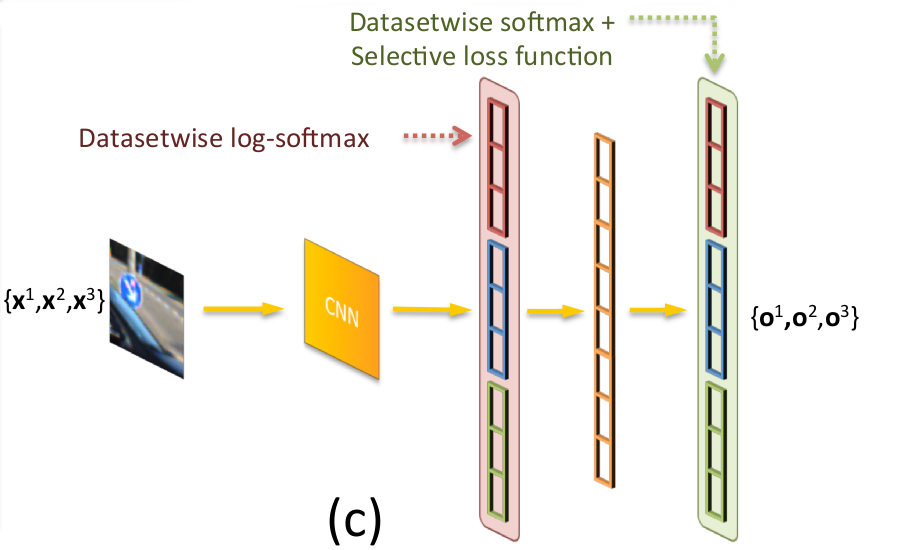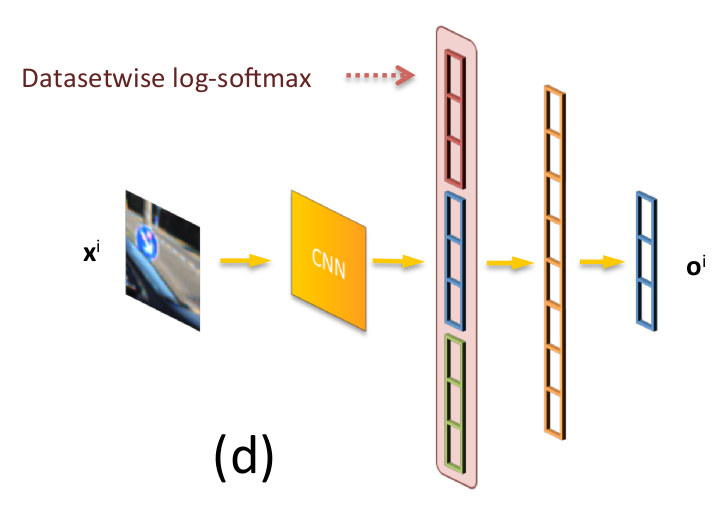## Joint Training − Results @svg:cnn-context/kitti-results.svg 800px 300px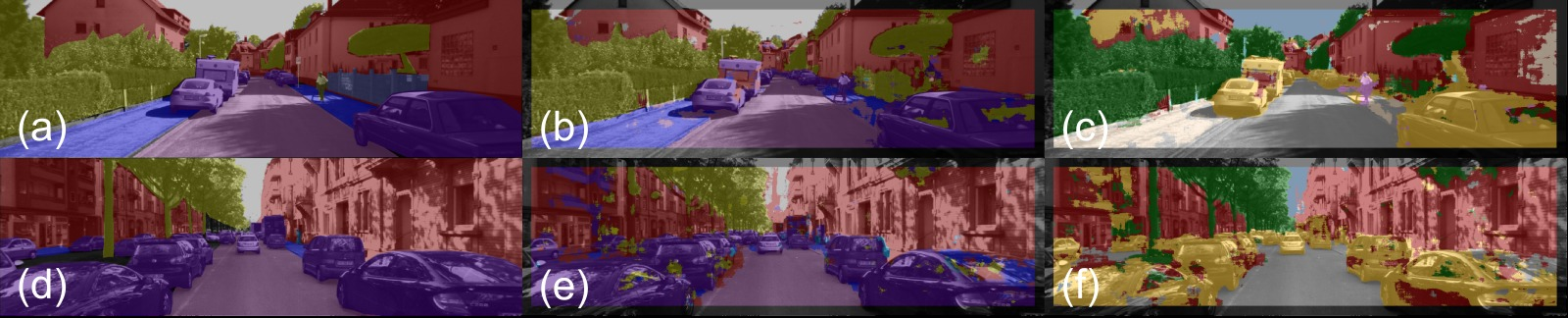# @copy:#plan # Thanks! More Questions?